## TWI (I2C) interface on AVR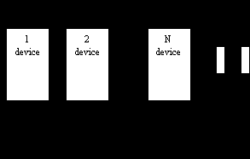Two-wire serial interfaces are included in the following AVR microcontroller families: ATmega8x, ATmega16x, ATmega163x, ATmega32x, ATmega323x, ATmega64x, and ATmega128x. TWI interface is a “Philips” standard I2C. Using the TWI interface, you can connect up to 128 devices using only two wires: clock (SCL) and data (SDA). Only two pull-up resistors on each line are needed for this interface to work properly. The I2C interface circuit is an open collector. This means if one of all devices has a low-level signal on a line, then it is ‘0’, and if all devices have a high impedance state, then the signal is considered to be great ‘1’—more details about TWI interface you can find on any ATmega datasheet. One of my examples Interfacing AD7416 digital temperature sensor you can find here: Analog Devices Digital temperature sensor AD7416

## Control 7 segment LED displays with AVR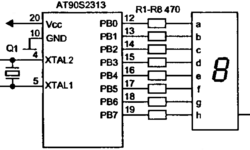LED displays are nothing more than sets of Light Emitting Diodes. The difference is that they have different shapes to display specific information. So driving LED displays is the same as regular LEDs. This is a simple connection when there are enough microcontroller Pins. But if you want to connect more displays, you will need more microcontroller pins than it can give you. Then it would help if you made a more advanced circuit with dynamic control.

## Drive relay with AVR microcontroller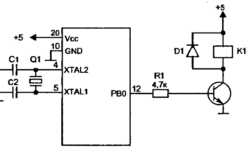To drive the relay, you need more than 20mA – the current can help one pin drive. This is why you cannot connect the relay directly to the pin of the microcontroller. To drive the relay, you need to connect a simple amplifier made of one transistor. One important part of this circuit is the diode, which protects the circuit from induction caused when switching the relay.

## Drive LED or Opto-isolators with AVR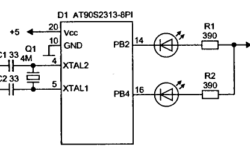This might seem very simple to many of you, but I still get questions about simple microcontroller interfacing. So I will put a thread of notes about interfacing AVR microcontrollers to devices like LED’s, relays, I2C, etc. As you might know, Diodes require a pretty small current. This current depends on diode type and can be from 3mA up to 20mA and more. Working voltage is from 1.5 to 4V. One AVR pin can sink up to 20mA of current; it is convenient to connect the diode directly to it with a current limiting resistor. Never connect the diode to the pin without a resistor – you may damage your AVR as your current may exceed the 20mA limit!

## K-Type Thermocouples reviewed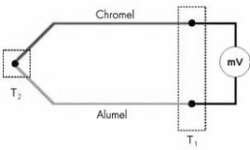A thermocouple is a sensor that generates an electrical potential related to the temperature. The sensor’s operating principle is based on the fact that any electrical junction between two different metals generates an electrical potential that depends on the temperature and the metals used. The principle applies equally well if three metals are used. In that case, there are two junctions in series and the net potential results from the series addition of the two individual potentials. For example, if a copper iron junction is in series with an iron-tin junction, the net potential is the same as for a copper-tin junction. However, that is only true if both junctions are at the same temperature. The K-Type thermocouple is usually made of Chromel (+) and Alumel(-). The voltage generated from this sensor is 4mV/100°C. The max temperature that can thermocouple withstand is 1000°C without any damage.

## Gauss-Zeidel optimization routines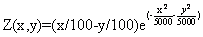This is the simplest optimization routine. Using this algorithm, optimization parameters are changed separately in each step. Only one parameter can be changed in one step while others are held as constants. Xk+1=Xk+ΔXk , k=0,1,2,… ΔXk step of parameter Xk. The parameter is changed until function growth is noticed, and then the next parameter follows, and so on. After the cycle with all parameters is completed, the step is changed to half its value and repeats. Optimal point searching ends when there is no function increase, and the last point is held as an optimal point. First function optimization example Its plot: Using the MATLAB script, we get the results below. In each picture, the start coordinates are different.

## Impulse signal distortion in the transmission line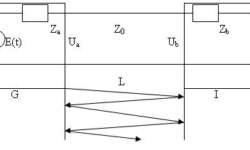Today most electronic equipment consists of signal generators and processing units. These units are connected with transmission lines. These lines have a big influence on signal distortions. On these lines depends transmission lines stability. Let’s see how transmission lines affect transmitted signals. (G- signal generator; Za– output impedance; I- signal receiver Zb– input impedance; L- transmission line length; Z0– Line impedance. When line is tuned and without losses then input voltage: Ub(t) = ZbE(t-t)/(Za + Zb) E(t)- generators signal amplitude; t- signal delay in line. t =l/v ; l- line lenght; v- signal speed. If the line is not tuned up, then there are distortions in line because of reflections inline: If signal E(t) is step function: Then signal in line exit in discrete time moments will be: U(0) = p; U(t) = p*(1+pb) U(2t) = p*(1+pb+pa*pb) U(3t) = p*(1+pb+pa*pb+pa*pb^2); U(4t) = p*(1+pb+pa*pb+pa*pb^2+pa^2*pb^2); U(5t) = p*(1+pb+pa*pb+pa*pb^2+pa^2*pb^2+pa^2*pb^3) â€¦ where Depending on reflectance coefficients and their signs distortions can differentiate or integrate: A real model using MathCAD was implemented to see how the signal looks on exit depending on parameters. Below you see used algorithm structure used in modeling: Part 2: One of results using trapezoid signal: In exit we get distorted…

## Life-giving to Atmega8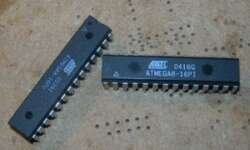I have got two ATmega microcontrollers from my friend. He stated that they are burned and can be thrown into the garbage. He also mentioned that they stopped responding after they were programmed. So I asked him to give those to me to try them. I had in my mind that this is a result of a bad usage of security bits. There is always confusion in these bits because of writing ‘0’ values program security bits and unprogrammed with ‘1’. I didn’t really expect to make them working again as my friend did quite rude experiments with them. What can I say – he was right by saying they are burned. But I guess some of you will like to see what I was doing to recover them. I decided that he unprogrammed all four security bits ( CKSEL0, CKSEL1, CKSEL2, CKSEL3 )by writing ‘1’ to them. This situation means that Atmega8 has to be clocked from an external clock signal. I was supposed to program those bits to work ATmega normally with a quartz resonator.

## Filter output dependency on tolerance of elements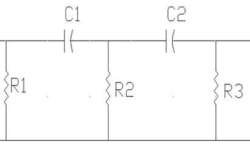Sometimes we need to project a simple filter with particular characteristics. We usually take calculations with ideal parameters and don’t look at tolerances of them. Let us see how simple filter output depends on its elements tolerances. For this let’s choose simple filter circuit: We are going to calculate the filter response characteristics. The bandpass frequency is taken on 0.707 level of response. We will see how this frequency depends on the electronic element tolerances. I will model elements with tolerances ±10%.